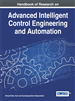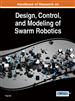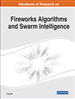Search the World's Largest Database of Information Science & Technology Terms & Definitions
InfScipedia
A Free Service of IGI Global Publishing House
Below please find a list of definitions for the term that
you selected from multiple scholarly research resources.

# What is Kalman FilterAlgorithm developed to estimate the future stateof a dynamic systemin an optimal way, depending on the delay of the samples admitted minimizing the noise.
Published in Chapter:
Maximum Likelihood-Based Fuzzy Adaptive Kalman Filter Applied to State Estimation of Permanent Magnet Synchronous Motors
Miriam M. Serrepe Ranno (Adaptive Systems and Signal Processing Laboratory, Federal University of Maranhão, São Luís, Brazil), Francisco das Chagas de Souza (Adaptive Systems and Signal Processing Laboratory, Federal University of Maranhão, São Luís, Brazil), and Ginalber L. O. Serra (Instituto Federal de Educação, Ciências e Tecnologia do Maranhão (IFMA), Brazil)
DOI: 10.4018/978-1-7998-2718-4.ch002
Abstract
In this chapter, a novel fuzzy adaptive Kalman filter for state estimation of a permanent magnet synchronous motor is proposed. The fuzzy set theory is used as a tool to perform on-line modification of the covariance matrices, adjusting the EKF and UKF parameters according to estimation reliability of the currents in the two windings of the rotor, position, and velocity for a two-phase permanent magnet synchronous motor. Also, the methodology uses the maximum likelihood technique, where the difference between the theoretical covariance and the measured covariance is defined as an approximation considering the average of a moving estimation window. This difference is performed continually and used to dynamically update the covariance matrices, aiming to obtain an efficient estimation. The membership functions are optimized to adjust the covariance matrices so that the error variation is minimal. Simulation results illustrate the efficiency and applicability of the proposed methodology.
More ResultsThe Kalman filter, also known as Linear Quadratic Estimation (LQE), is an algorithm for estimating the state variables of stochastic systems that are described by state-space mathematical models, based on experimental measurements.An algorithm that uses a series of measurements observed over time, containing statistical noise and other inaccuracies, and produces estimates of unknown variables that tend to be more accurate than those based on a single measurement alone, by using Bayesian inference and estimating a joint probability distribution over the variables for each timeframe. The filter is named after Rudolf E. Kálmán, one of the primary developers of its theory.An algorithm used to reduce the amount of noise in a stream of data.It is an algorithm usually used for state estimation problems. It delivers estimates of a system state based on inaccurate and uncertain measurements.Is dynamic adaptive filter the amplifier factor of which calculated from the Riccati differential equation using statistical information of signal and noise.